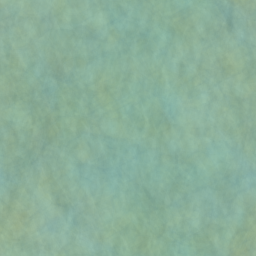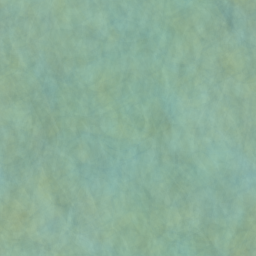Published August 1, 20209 minute read

How do you average (or sum) a lot of numbers, quickly?

More precisely, how do you do this on a webpage, preferably in real-time, when your values are stored as pixels in a large image? In general-purpose applications, we have access to the massive parallelization capabilities of GPUs with CUDA or OpenCL — but on the web, we’re stuck with WebGL and fragment shaders.

One possibility is to write a fragment shader that repeatedly downscales the image by a factor of 2, where each output pixel is the average of the four pixels in its preimage. Assuming each render takes constant time due to GPU parallelization, this technique can average an $n \times n$ texture in $\log_2{n}$ time.This works, but has a few disadvantages:

• It’s a pain to deal with images with sidelength not a power of 2. It can be done, but I wouldn’t want to do it.
• Each iteration requires a texture of a different size. Instantiating and swapping around all of these textures (or managing glViewport) is a pain.
• Perhaps most importantly, this technique clearly works and is thus mathematically uninteresting.

An alternative technique that “resolves” some of these issues is to just blur the image repeatedly until it becomes a solid color, then read an arbitrary pixel.This technique:

• Works equally well on images of any size or shape.
• Uses textures of all the same size, so we only need to instantiate two textures and render back and forth between them.
• And, as we’re about to see, is very interesting.

To obtain an accurate average and avoid edge-effects, we treat the texture as a torus. What the blur operation is really doing, then, is circularly convolving the input image with a Gaussian kernel:$I_0$

$*$$K$

$=$$I_1 = I_0 * K$

As you may have guessed from the above images, however, repeated Gaussian blurring is really bad for this purpose. The issue is that the Gaussian kernel is very local, so information doesn’t spread very fast throughout the image.

## How to Judge a Kernel

Perhaps a kernel that’s more spread out would do better — indeed, there’s no reason for the kernel to even be contiguous!

But first, we need a way to quantify the “quality” of a kernel $K$. Let $I_0$ denote the original image, and let $I_n$ denote the image after convolving with $K$ a total of $n$ times. Then $I_n = I_{n-1} * K$, and taking the 2D discrete Fourier transform yields $\hat{I}_n = \hat{I}_{n-1} \cdot \hat{K}$.From here, we easily obtain $\hat{I}_n = \hat{I}_0 \cdot \hat{K}^n$.

The image $I_\infty$ we want to eventually converge to has all pixels equal to the average value $\langle I_0 \rangle$ of $I_0$, so that $\hat{I}_\infty(0, 0) = \hat{I}_0(0, 0)$ is the only non-zero coefficient in $\hat{I}_\infty$. Intuitively, the “quality” of the kernel $K$ should quantify how quickly the $I_n \to I_\infty$, say in $\ell^2$ norm. By Parseval’s theorem, it is equivalent to study the convergence of $\hat{I}_n \to \hat{I}_\infty$ in $\ell^2$. To do this, we’ll first need to deduce a bit more about $\hat{K}$.

Let $e_{i, j}$ for $0 \leq i, j \leq N$ denote the image with value 1 at pixel $(i, j)$ and 0 elsewhere. Then every coefficient in $\hat{e}_{i, j}$ has magnitude 1, by definition.If we assume that the kernel $K$ is a convex linear combination of the $e_{i, j}$, then every coefficient in its Fourier transform $\hat{K}$ is a convex linear combination of norm-1 complex numbers — and thus has norm at most 1. Thus all components of $\hat{I}_n$ either decay exponentially to zero or remain fixed as $n \to \infty$.

In fact, since all $\hat{e}_{i, j}(0, 0) = 1$, we have $\hat{K}(0, 0)$ = 1, and so all $\hat{I}_n(0, 0) = \hat{I}_\infty(0, 0)$. Letting $K'$ denote the kernel $K$ with the entry $(0, 0)$ set to zero, we finally define the quality of $K$ to be

$Q(K) \defeq \frac{1}{\ell^\infty(\hat{K'})}.$

Put simply, we look at the largest coefficient in $\hat{K}$ by magnitude, excluding $\hat{K}(0, 0)$. Then the rate of convergence of $\hat{I}_n \to \hat{I}_\infty$ is on the order of $Q(K)^{-n}$: our average becomes roughly $Q(K)$ times better on each iteration.

Sidenote: We can now understand precisely why the Gaussian kernel is so bad: the magnitude of the Fourier transform of a Gaussian is a Gaussian, which has a very “flat” peak near $(0, 0)$. Thus the Fourier coefficients near $(0, 0)$ have magnitudes almost exactly equal to 1.

## The Search Begins

We now have a clearly defined task: find a kernel $K$ of maximal quality $Q(K)$.

Clearly, the optimal quality is $\infty$, when all coefficients of $\hat{K}$ other than $\hat{K}(0, 0)$ are zero. This corresponds to a filter with equal weight on every pixel of the image, so we converge in one step. End of post!

Of course, in practice this would be horrendously slow, even on the GPU. The performance of our fragment shader is related to the number of input pixels we access per output pixel — i.e., the number of non-zero entries of $K$. So, our task is really:

Find a kernel $K$ of maximal quality $Q(K)$, subject to $\ell^0(K) \leq N$.

There’s just one issue: this task is horrendously difficult.

This forces us to turn to brute-force Monte-Carlo methods. The procedure I used is as follows:

1. Randomly choose $N$ pixels without replacement from the $n \times n$ input image. These locations represent precisely the non-zero entries of $K$.
2. Given this restriction, maximize $Q(K)$ using the method of your choice. This is an $N$-dimensional optimization problem where the variables must be non-negative and sum to one. I used scipy.optimize.
3. Repeat.

Keeping track of the maximum-quality kernel during this procedure should give a good estimate of the true solution. Here’s some experimental results for a square image of sidelength $n =128$:As expected, the quality increases as the sparsity budget $N$ is increased. For a given $N$, the median kernel quality, in the grand scheme of things, isn’t that much worse than that of the best observed candidate.

So perhaps we don’t need to find the absolute best kernel for a given sparsity; using randomly chosen pixel locations yields comparable quality, and we can just increase $N$ by a bit if it’s not good enough.

## Theoretical Insight

How can we understand this result theoretically? The optimization step (Step 2) is quite difficult to think about, so let’s assume that all $N$ randomly chosen pixels end up with equal weights.

Consider an arbitrary coefficient $\hat{K}(u, v)$, excluding $(u, v) = (0, 0)$. This coefficient will be the average of $\hat{e}_{i, j}(u, v)$ for $N$ randomly chosen pairs $(i, j)$. Since $\hat{e}_{i, j}(u, v)$ has unit norm and essentially random phase, its real part has a mean of zero and a variance of$\frac{1}{2\pi}\int_0^{2\pi} \cos^2{\theta} \, \dd{\theta} = \frac{1}{2}.$Assuming the $(i, j)$ are roughly independent and $N$ is relatively large, we have $\mathrm{Re}(\hat{K}(u, v)) \sim \mathcal{N}(0, 1/(2N))$ by the Central Limit Theorem. The same holds for the imaginary component, so $\abss{\hat{K}(u, v)}$ has the Rayleigh distribution$\abss{\hat{K}(u, v)} \sim \frac{x}{\sigma^2} e^{-x^2/(2 \sigma^2)}, \quad x \geq 0,$where $\sigma^2 = 1/(2N)$.

Since the kernel $K$ is real-valued, half of its Fourier coefficients will be redundant, so we may treat $M \defeq \ell^\infty(\hat{K}')$ as the maximum of $n^2/2$ independent Rayleigh-distributed random variables. The CDF of each Rayleigh-distributed variable is $F(x) = 1-e^{-x^2 / (2 \sigma^2)}$, so the expected value of the maximum is$\mathbb{E}(M) = \int_0^\infty (1 - F(x)^{n^2/2}) \, \dd{x}.$Assuming $N$ and $n^2$ are both fairly large, the integrand can be well-approximated as a step function that transitions from $1$ to $0$ at the $x$-value satisfying $F(x)^{n^2/2} = \frac{1}{2}$. Solving, we obtain

\begin{aligned} \mathbb{E}(M) &\approx \sigma \sqrt{-2 \log(1 - \sqrt[n^2/2]{1/2})} \\ &\approx \sqrt{\frac{2\log{n} - \log{2} - \log{\log{2}}}{N}}. \end{aligned}

The median quality $Q(K)$ should be roughly the inverse of this. With fingers crossed, we compare the theory to experiment:Not bad, but not great. The actual median $Q(K)$ is a bit higher, presumably due to the optimization step (Step 2) that we overlooked. Indeed, if we disable the optimization step and weight each kernel entry equally, the results match quite closely:Thus we can expect our estimate to provide a reliable lower bound for the optimized median. This is actually great news: even randomly choosing pixels and setting equal weights, to achieve a target quality $Q$, we need only choose a filter with a size of

$N \approx 2 Q^2 \log{n},$

which grows only logarithmically with the image size! Thus, even for fairly large images, the kernel remains sparse enough for near-constant-time evaluation on the GPU.

## Demo Time!

The example image I’ve been using has sidelength $n = 256$. Aiming for a quality of $Q = 4$, our formula tells us we need to choose a random kernel with $N = 178$ entries. Here’s the results with no kernel optimization (equal weights):Here’s the results after optimizing the kernel (Step 2 in the procedure described before):Yep. Even with the unoptimized kernel, after two iterations the average is resolved to a resolution of at least $256 / Q^2 = 16$ in each channel, which is barely perceptible. For comparison, the Gaussian kernel I used at the beginning of the post had $101 \times 101 = 10,201$ entries.

To achieve the same result with the scaling-based algorithm outlined at the start of the post, you would need to scale down by $16 \times$ at each step, so each output pixel would need to look at $256$ input pixels. Admittedly, this isn’t a direct comparison, since the scaling method provides an exact solution — but for many applications, a rapidly converging approximate solution does the job.

And finally, for fun, a demo that calculates the value of $\pi$ by averaging an image of a circle! The estimate shown is derived from a single pixel in the displayed image.

$\pi \approx 0.00000...$

Please enable Javascript and WebGL to use the demo.

Kernel Sparsity

$N = 50$

Texture Size: 300 × 300
Estimated Quality: Q(K) = 2.094

## Footnotes

1. Assuming we’re hitting up against the 60 FPS frame cap, this is a reasonable assumption.
2. Incidentally, the glGenerateMipmap function executes essentially this procedure — and is restricted to power-of-two sidelengths only. WebGL 2 supports non-power-of-two textures, but there’s no guarantee the edge effects will be treated as to give an accurate average...
3. Here, we use $\cdot$ to denote component-wise multiplication.
4. This also defines the convention we’re using for our Fourier transforms.
5. This is a reasonable assumption — the components should sum to one to preserve the average on each iteration. You can convince yourself (fun exercise!) that negative components will lead to exponential blowups in certain situations.
6. To the best of my knowledge, anyway. If anyone manages to find a reference, I’d love to hear about it!
7. So really, there are $N-1$ degrees of freedom.
8. There is some theoretical basis to this assumption — choosing all weights equal minimizes the $\ell^2$ norm of $K$, which (by Parseval’s theorem) minimizes the $\ell^2$ norm of $\hat{K}'$. The $\ell^2$ norm is an upper bound for the $\ell^\infty$ norm we really care about.
9. As with many of the assumptions here, this isn’t strictly true, but works well enough.
10. Since the Gaussian kernel can be factored, this really only needs 2 passes, one horizontal and one vertical, with a kernel of size 101. But even then, the results with the Gaussian are objectively much worse.
11. For fun, you can try to figure out what kernel I used by downloading the first two images, dividing their 2D discrete Fourier transforms, and taking an inverse DFT. I haven't tried this and have no idea if it will work (since the pixel colors are quantized).
12. Through some clever WebGL hacks, I am using all four color channels internally to increase the precision. There also appears to be a bug on mobile (possibly due to low precision?) that causes a small bit of drift in the final average.